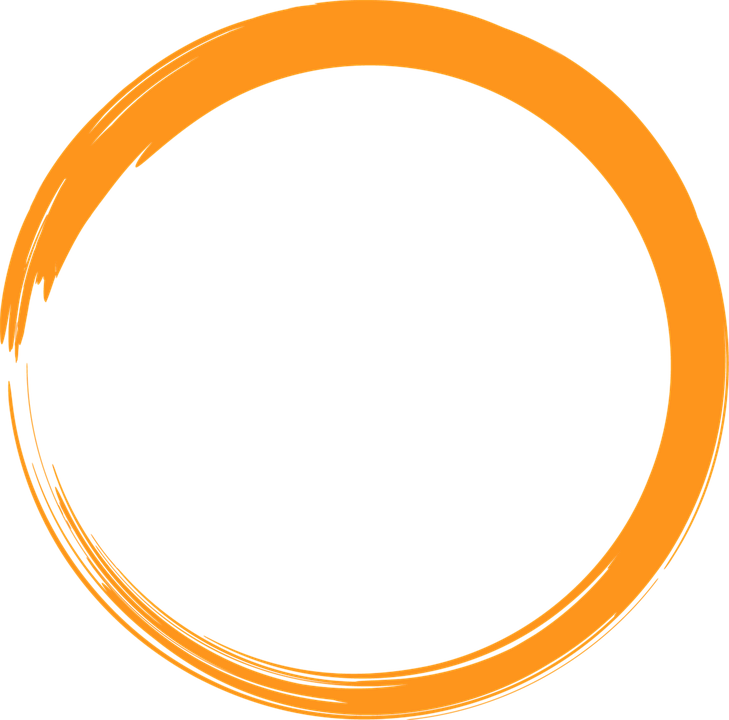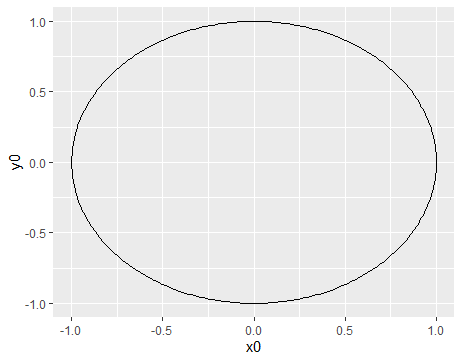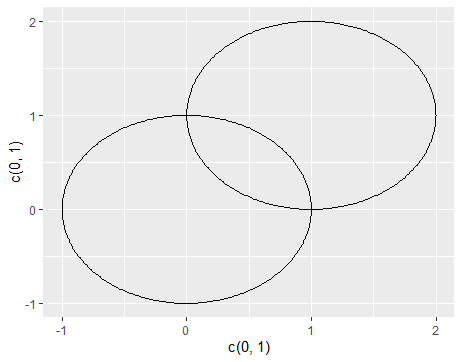# Plotting A Circle In R

in STEMGeeks2 months ago

Hi there. This a quick post on plotting a circle in the R programming language.

I wanted to plot a circle as part of a personal project I am working on. Here is what I found.Pixabay Image Source

## Plotting A Single Circle With ggforce and geom_circle()

With the regular `ggplot2` package for data visualization in R there is no command for drawing circles. I have tried using stat_function and geom_function but those are for rectangles and probability distribution curves.

I found this Stack Overflow thread on this matter. The last comment mentions about the `ggforce` package and the `geom_circle()` function. I decided to test it out.

To plot a circle in R with `ggforce` is not to bad actually. The hard part is understanding the arguments in the command.

``````# Load packages (after installation)
library(ggplot2)
library(ggforce)

# Plotting a circle centered at (0, 0) with radius 1
ggplot() +
geom_circle(aes(x0 = 0, y0 = 0, r = 1))

``````The circle in `geom_circle()` is centered at (x0, y0) with a radius 1 (diameter = 2).

The x0 and y0 arguments can also take in R vector of numbers. (In R, use`c()` to create a vector)

``````# Multiple circles: First centered at (0, 0), second one centered at (1, 1)
ggplot() +
geom_circle(aes(x0 = c(0, 1), y0 = c(0, 1), r = 1))

``````These two circles both have a radius of 1 but have different centers. The first (left) circle has a center of (0, 0) and the upper right second circle has a center of (1, 1).

In the R code, the first value in the x0 vector refers to the first 0 in center (0, 0) and the second value in the x0 vector refers to the first 1 in center (1, 1). The second value in x0 refers to the second 0 in center (0, 0) and the second value in y0 refers to the second value in the circle center (1, 1).

Yes, the labels need fixing but that can be done easily with adding `labs()` and its arguments.

RDocumentation On `geom_circle()`: https://www.rdocumentation.org/packages/ggforce/versions/0.3.2/topics/geom_circle

https://stackoverflow.com/questions/6862742/draw-a-circle-with-ggplot2